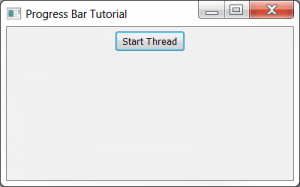# wxPython: How to Update a Progress Bar from a Thread

Every now and then, I see someone wondering how to create a progress bar and update it. So I decided to whip up an example application that updates a progress bar (technically a wx.Gauge widget) from a thread. In this tutorial, we will create a frame with a button. When the button is pushed, it will launch a dialog that contains our progress bar and it will start a thread. The thread is a dummy thread in that it doesn’t do anything in particular except send an update back to the dialog once a second for twenty seconds. Then the dialog is destroyed. Let’s take a look!

```import time
import wx

from wx.lib.pubsub import Publisher

########################################################################

#----------------------------------------------------------------------
def __init__(self):

#----------------------------------------------------------------------
def run(self):
# This is the code executing in the new thread.
for i in range(20):
time.sleep(1)
wx.CallAfter(Publisher().sendMessage, "update", "")

########################################################################
class MyProgressDialog(wx.Dialog):
""""""

#----------------------------------------------------------------------
def __init__(self):
"""Constructor"""
wx.Dialog.__init__(self, None, title="Progress")
self.count = 0

self.progress = wx.Gauge(self, range=20)

sizer = wx.BoxSizer(wx.VERTICAL)
self.SetSizer(sizer)

# create a pubsub listener
Publisher().subscribe(self.updateProgress, "update")

#----------------------------------------------------------------------
def updateProgress(self, msg):
"""
Update the progress bar
"""
self.count += 1

if self.count >= 20:
self.Destroy()

self.progress.SetValue(self.count)

########################################################################
class MyFrame(wx.Frame):

#----------------------------------------------------------------------
def __init__(self):
wx.Frame.__init__(self, None, title="Progress Bar Tutorial")

# Add a panel so it looks the correct on all platforms
panel = wx.Panel(self, wx.ID_ANY)
self.btn = btn = wx.Button(panel, label="Start Thread")
btn.Bind(wx.EVT_BUTTON, self.onButton)

sizer = wx.BoxSizer(wx.VERTICAL)
panel.SetSizer(sizer)

#----------------------------------------------------------------------
def onButton(self, event):
"""
"""
btn = event.GetEventObject()
btn.Disable()

dlg = MyProgressDialog()
dlg.ShowModal()

btn.Enable()

#----------------------------------------------------------------------
# Run the program
if __name__ == "__main__":
app = wx.App(False)
frame = MyFrame()
frame.Show()
app.MainLoop()
```

Let’s spend a few minutes breaking this down. We’ll start at the bottom. The MyFrame class is what gets run first. When you run this script you should see something like this:As you can see, all this code does is create a simple frame with a button on it. If you press the button, the following dialog will be created and a new thread will start:Let’s look at the portion of the code that makes the dialog:

```########################################################################
class MyProgressDialog(wx.Dialog):
""""""

#----------------------------------------------------------------------
def __init__(self):
"""Constructor"""
wx.Dialog.__init__(self, None, title="Progress")
self.count = 0

self.progress = wx.Gauge(self, range=20)

sizer = wx.BoxSizer(wx.VERTICAL)
self.SetSizer(sizer)

# create a pubsub listener
Publisher().subscribe(self.updateProgress, "update")

#----------------------------------------------------------------------
def updateProgress(self, msg):
"""
Update the progress bar
"""
self.count += 1

if self.count >= 20:
self.Destroy()

self.progress.SetValue(self.count)
```

This code just creates a dialog with a wx.Gauge widget. The Gauge is the actual widget behind the progress bar. Anyway, we create a pubsub listener at the very end of the dialog’s __init__. This listener accepts messages that will fire off the updateProgress method. We will see the messages get sent in the thread class. In the updateProgress method, we increment the counter and update the wx.Gauge by setting its value. We also check to see if the count is greater than or equal to 20, which is the range of the gauge. If it is, then we destroy the dialog.

```########################################################################

#----------------------------------------------------------------------
def __init__(self):

#----------------------------------------------------------------------
def run(self):
# This is the code executing in the new thread.
for i in range(20):
time.sleep(1)
wx.CallAfter(Publisher().sendMessage, "update", "")

```

Here we create a thread and immediately start it. The thread loops over a range of 20 and uses the time module to sleep for a second in each iteration. After each sleep, it sends a message to the dialog to tell it to update the progress bar.

### Updating the Code for wxPython 2.9

The code in the previous section was written using pubsub’s old API which has been tossed out the window with the advent of wxPython 2.9. So if you try to run the code above in 2.9, you will likely run into issues. Thus for completeness, here is a version of the code that uses the new pubsub API:

```import time
import wx

from wx.lib.pubsub import pub

########################################################################

#----------------------------------------------------------------------
def __init__(self):

#----------------------------------------------------------------------
def run(self):
# This is the code executing in the new thread.
for i in range(20):
time.sleep(1)
wx.CallAfter(pub.sendMessage, "update", msg="")

########################################################################
class MyProgressDialog(wx.Dialog):
""""""

#----------------------------------------------------------------------
def __init__(self):
"""Constructor"""
wx.Dialog.__init__(self, None, title="Progress")
self.count = 0

self.progress = wx.Gauge(self, range=20)

sizer = wx.BoxSizer(wx.VERTICAL)
self.SetSizer(sizer)

pub.subscribe(self.updateProgress, "update")

#----------------------------------------------------------------------
def updateProgress(self, msg):
""""""
self.count += 1

if self.count >= 20:
self.Destroy()

self.progress.SetValue(self.count)

########################################################################
class MyForm(wx.Frame):

#----------------------------------------------------------------------
def __init__(self):
wx.Frame.__init__(self, None, wx.ID_ANY, "Tutorial")

# Add a panel so it looks the correct on all platforms
panel = wx.Panel(self, wx.ID_ANY)
self.btn = btn = wx.Button(panel, label="Start Thread")
btn.Bind(wx.EVT_BUTTON, self.onButton)

sizer = wx.BoxSizer(wx.VERTICAL)
panel.SetSizer(sizer)

#----------------------------------------------------------------------
def onButton(self, event):
"""
"""
btn = event.GetEventObject()
btn.Disable()

dlg = MyProgressDialog()
dlg.ShowModal()

btn.Enable()

#----------------------------------------------------------------------
# Run the program
if __name__ == "__main__":
app = wx.App(False)
frame = MyForm().Show()
app.MainLoop()
```

Note that now you import the pub module rather than the Publisher module. Also note that you have to use keyword arguments. See the pubsub documentation for additional information.